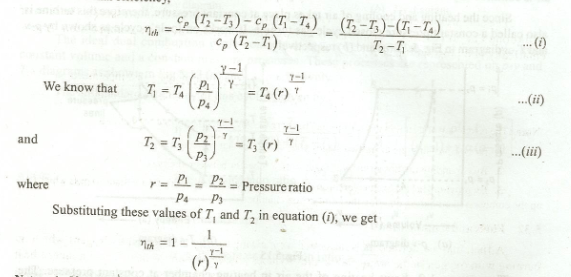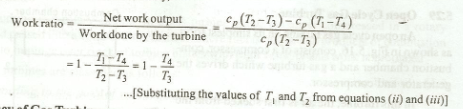# Thermal Efficiency of Ideal Gas Turbine Plant

Thermal Efficiency of Ideal Gas Turbine Plant is defined as the ratio of net work output obtained from the plant to the total heat supplied. The p-v and T-s diagram for an ideal gas turbine working on Joule cycle is shown in Fig 5.15.

We know that net work output obtained from the plant

= Workdone by the turbine - Workdone by compressor

= Cp (T2 -T3)-Cp (T1 -T4 )

and

heat supplied = Cp (T2 -T1)

Therefore Thermal efficiency,Notes:

1. If the heat supplied is obtained from the mass of fuel x Calorific value, then the corresponding efficiency will be overall efficiency.

2. The ratio of the net work output obtained from the plant to the workdone by the turbine, is known as work ratio of the turbine plant. Mathematically,